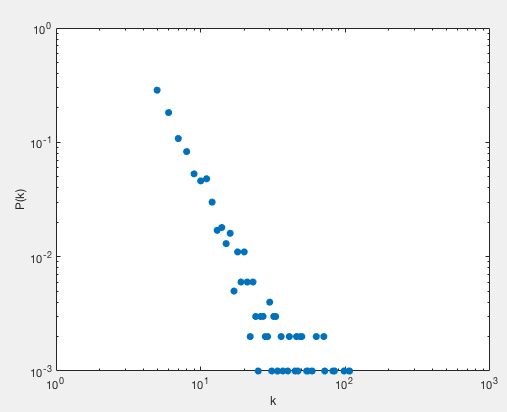# 复杂网络-无标度网络matlab代码实现

    1。网络是通过新增节点而不断扩展的。
2。新增的节点会优先连接在连通性更好的节点上。


1。先创建一个小型随机网络
2。新增节点，并且优先连接在度更大的节点上。

%随机连边  1表示有边
for i=1:m0
for j= (i+1):m0
A(i,j)= round(rand());   %非1即0
A(j,i) =  A(i,j);
end
end   %初始完成


3个集合sum 一下 ，得到 2，2，1 ，这个就是每个度的个数了。2/（2+2+1） 就是度的概率了。clear all;
tic
m = 5;    %每次加入的边个数
m0 = 5    %初始的顶点个数
N = 1000;   %最终达到的顶点个数

%创建邻接矩阵    全0，无边
A = sparse(N,N);

%随机连边  1表示有边
for i=1:m0
for j= (i+1):m0
A(i,j)= round(rand());
A(j,i) =  A(i,j);
end
end   %初始完成

%加入的节点为 A(new) , 与其连接的点为old     生成 A(new ， old)
for new = m0+1:N
new
%old vertice 度越大连接上的概率越大
Degree = sum(A(1:new-1,1:new-1));  %每个顶点的度
%制造出一个度的分布区间，模拟概率
DegreeInterval(1) = Degree(1);
for i=2:new-1
DegreeInterval(i) = Degree(i)+DegreeInterval(i-1);
end
%连接 新节点 与 m个old节点
AllDegree = sum(sum(A(1:new-1,1:new-1))); %整个图的总度
for i = 1:m
while 1
%以概率从old节点中找到合适的顶点连接
RandDegree  = fix(AllDegree*rand()+1); %要与度区间包含RandDegree的顶点相连
%找到 符合 要求的区间所属顶点
Ans = find(RandDegree <= DegreeInterval(1:new-1));
old = Ans(1);
if A(new,old) == 0
A(new,old) = 1;
A(old,new) = 1;
break;         %成功连接
end
end
end
end
%求度分布
Degree = sum(A);  %完成后的网络的每个节点的度  2 3 2 2 4 3
UniDegree = unique(Degree);  %去重后度       2 3 4
for i = 1:length(UniDegree)
DegreeNum(i) = sum(Degree==UniDegree(i));
end
toc
%画图
loglog(UniDegree, DegreeNum ./ sum(DegreeNum),'.','markersize',18)
xlabel('k'),ylabel('P(k)')


04-043241

#### 复杂网络实验3：BA模型（matlab）

05-078187

#### 【复杂网络学习笔记】2：无标度网络的建立01-195576

#### 复杂网络之无标度网络与小世界网络生成程序

05-192万+

#### BA无标度网络模型构造算法

12-138761

#### 012 MATLAB复杂网络处理入门

09-157245

#### 复杂网络-小世界网络的matlab实现过程

04-01602

#### 复杂网络matlab学习记录（1）2019年4月1日

03-285420

#### 复杂网络实验1：ER随机图（matlab）

10-27414

#### 复杂网络多局域世界模型matlab算法实现

10-111万+

#### 复杂网络介绍（一）

11-281555

#### 复杂网络之无标度网络的界限©️2020 CSDN 皮肤主题: 大白 设计师: CSDN官方博客点击重新获取扫码支付1.余额是钱包充值的虚拟货币，按照1:1的比例进行支付金额的抵扣。
2.余额无法直接购买下载，可以购买VIP、C币套餐、付费专栏及课程。余额充值